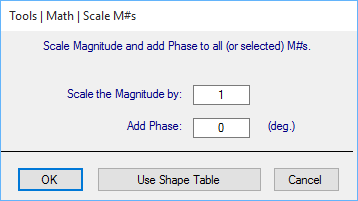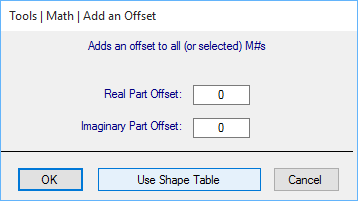## Math | Scale M#s

Multiplies all (or selected) M#s by a magnitude and adds phase to them.• The Use Shape Table button scales each M# with a different scale factor from a Shape Table.

• The magnitude & phase of each M# of the first shape in the Shape Table is used to scale each matching M# in the Data Block

## Math | Add an Offset

Adds a complex (real & imaginary) offset to all (or selected) M#s.• The Use Shape Table button adds a different offset from a Shape Table to each M#.

• The complex value for each M# of the first shape in the Shape Table is used to offset each matching M# in the Data Block.

## Math | Add Random Noise

Adds random noise to each M#.  The amount of random noise is a percentage of the maximum magnitude of each M#.

## Math | Conjugate Traces

Replaces each complex measurement value with its complex conjugate.  The Real part remains the same and the Imaginary part is multiplied by "-1".

## Math | Invert M#s

Replaces each M# with its inverse.

## Math | Square M#s

Replaces each M# with its value squared.

## Math | Square Root of M#s

Replaces each M# with the square root of its value.

## Math | Smooth M#s

Performs multi-point smoothing of each measurement in a Data Block.  Replaces each measurement sample with the average value of N samples of data surrounding that sample.

For example, for N = 3,

M# (sample) = (M# (sample) + M# (sample-1) + M# (sample +1) ) / 3

## Math | Re-Sample M#s

Changes the number of samples in a Data Block without changing the X-Axis span by re-sampling the measurements

## Math | Sum M#s

Sums all (or selected) M# values at each sample and stores the result into a single M#.

## Math | Average M#s

Divides the sum of all (or selected) M# values at each sample by the number of M#s, and stores the result into a single M#.

## Math | Add (Subtract, Multiply by, Divide by) a selected M#

Performs the indicated operation between the M#s in a Data Block and a selected M# in the same of a different Data Block..

If two Data Blocks have the same number of M#s in them, you can choose to perform the operation between M# pairs in the two Data Blocks.